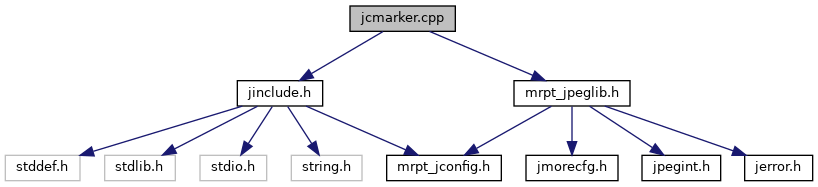Main MRPT website > C++ reference for MRPT 1.5.6
jcmarker.cpp File Reference
`#include "jinclude.h"`
`#include "mrpt_jpeglib.h"`
Include dependency graph for jcmarker.cpp:Go to the source code of this file.

Classes

struct  my_marker_writer

Macros

#define JPEG_INTERNALS

Typedefs

typedef my_marker_writermy_marker_ptr

Enumerations

enum  JPEG_MARKER {
M_SOF0 = 0xc0, M_SOF1 = 0xc1, M_SOF2 = 0xc2, M_SOF3 = 0xc3,
M_SOF5 = 0xc5, M_SOF6 = 0xc6, M_SOF7 = 0xc7, M_JPG = 0xc8,
M_SOF9 = 0xc9, M_SOF10 = 0xca, M_SOF11 = 0xcb, M_SOF13 = 0xcd,
M_SOF14 = 0xce, M_SOF15 = 0xcf, M_DHT = 0xc4, M_DAC = 0xcc,
M_RST0 = 0xd0, M_RST1 = 0xd1, M_RST2 = 0xd2, M_RST3 = 0xd3,
M_RST4 = 0xd4, M_RST5 = 0xd5, M_RST6 = 0xd6, M_RST7 = 0xd7,
M_SOI = 0xd8, M_EOI = 0xd9, M_SOS = 0xda, M_DQT = 0xdb,
M_DNL = 0xdc, M_DRI = 0xdd, M_DHP = 0xde, M_EXP = 0xdf,
M_APP0 = 0xe0, M_APP1 = 0xe1, M_APP2 = 0xe2, M_APP3 = 0xe3,
M_APP4 = 0xe4, M_APP5 = 0xe5, M_APP6 = 0xe6, M_APP7 = 0xe7,
M_APP8 = 0xe8, M_APP9 = 0xe9, M_APP10 = 0xea, M_APP11 = 0xeb,
M_APP12 = 0xec, M_APP13 = 0xed, M_APP14 = 0xee, M_APP15 = 0xef,
M_JPG0 = 0xf0, M_JPG13 = 0xfd, M_COM = 0xfe, M_TEM = 0x01,
M_ERROR = 0x100, M_SOF0 = 0xc0, M_SOF1 = 0xc1, M_SOF2 = 0xc2,
M_SOF3 = 0xc3, M_SOF5 = 0xc5, M_SOF6 = 0xc6, M_SOF7 = 0xc7,
M_JPG = 0xc8, M_SOF9 = 0xc9, M_SOF10 = 0xca, M_SOF11 = 0xcb,
M_SOF13 = 0xcd, M_SOF14 = 0xce, M_SOF15 = 0xcf, M_DHT = 0xc4,
M_DAC = 0xcc, M_RST0 = 0xd0, M_RST1 = 0xd1, M_RST2 = 0xd2,
M_RST3 = 0xd3, M_RST4 = 0xd4, M_RST5 = 0xd5, M_RST6 = 0xd6,
M_RST7 = 0xd7, M_SOI = 0xd8, M_EOI = 0xd9, M_SOS = 0xda,
M_DQT = 0xdb, M_DNL = 0xdc, M_DRI = 0xdd, M_DHP = 0xde,
M_EXP = 0xdf, M_APP0 = 0xe0, M_APP1 = 0xe1, M_APP2 = 0xe2,
M_APP3 = 0xe3, M_APP4 = 0xe4, M_APP5 = 0xe5, M_APP6 = 0xe6,
M_APP7 = 0xe7, M_APP8 = 0xe8, M_APP9 = 0xe9, M_APP10 = 0xea,
M_APP11 = 0xeb, M_APP12 = 0xec, M_APP13 = 0xed, M_APP14 = 0xee,
M_APP15 = 0xef, M_JPG0 = 0xf0, M_JPG13 = 0xfd, M_COM = 0xfe,
M_TEM = 0x01, M_ERROR = 0x100
}

Functions

emit_byte (j_compress_ptr cinfo, int val)

emit_marker (j_compress_ptr cinfo, JPEG_MARKER mark)

emit_2bytes (j_compress_ptr cinfo, int value)

emit_dqt (j_compress_ptr cinfo, int index)

emit_dht (j_compress_ptr cinfo, int index, boolean is_ac)

emit_dac (j_compress_ptr)

emit_dri (j_compress_ptr cinfo)

emit_sof (j_compress_ptr cinfo, JPEG_MARKER code)

emit_sos (j_compress_ptr cinfo)

emit_jfif_app0 (j_compress_ptr cinfo)

write_marker_header (j_compress_ptr cinfo, int marker, unsigned int datalen)

write_marker_byte (j_compress_ptr cinfo, int val)

write_file_trailer (j_compress_ptr cinfo)

write_tables_only (j_compress_ptr cinfo)

jinit_marker_writer (j_compress_ptr cinfo)

Macro Definition Documentation

 #define JPEG_INTERNALS

Definition at line 10 of file jcmarker.cpp.

Typedef Documentation

 typedef my_marker_writer* my_marker_ptr

Definition at line 91 of file jcmarker.cpp.

Enumeration Type Documentation

 enum JPEG_MARKER
Enumerator
M_SOF0
M_SOF1
M_SOF2
M_SOF3
M_SOF5
M_SOF6
M_SOF7
M_JPG
M_SOF9
M_SOF10
M_SOF11
M_SOF13
M_SOF14
M_SOF15
M_DHT
M_DAC
M_RST0
M_RST1
M_RST2
M_RST3
M_RST4
M_RST5
M_RST6
M_RST7
M_SOI
M_EOI
M_SOS
M_DQT
M_DNL
M_DRI
M_DHP
M_EXP
M_APP0
M_APP1
M_APP2
M_APP3
M_APP4
M_APP5
M_APP6
M_APP7
M_APP8
M_APP9
M_APP10
M_APP11
M_APP12
M_APP13
M_APP14
M_APP15
M_JPG0
M_JPG13
M_COM
M_TEM
M_ERROR
M_SOF0
M_SOF1
M_SOF2
M_SOF3
M_SOF5
M_SOF6
M_SOF7
M_JPG
M_SOF9
M_SOF10
M_SOF11
M_SOF13
M_SOF14
M_SOF15
M_DHT
M_DAC
M_RST0
M_RST1
M_RST2
M_RST3
M_RST4
M_RST5
M_RST6
M_RST7
M_SOI
M_EOI
M_SOS
M_DQT
M_DNL
M_DRI
M_DHP
M_EXP
M_APP0
M_APP1
M_APP2
M_APP3
M_APP4
M_APP5
M_APP6
M_APP7
M_APP8
M_APP9
M_APP10
M_APP11
M_APP12
M_APP13
M_APP14
M_APP15
M_JPG0
M_JPG13
M_COM
M_TEM
M_ERROR

Definition at line 15 of file jcmarker.cpp.

Function Documentation

 emit_2bytes ( j_compress_ptr cinfo, int value )

Definition at line 130 of file jcmarker.cpp.

References emit_byte().

Definition at line 383 of file jcmarker.cpp.

References emit_2bytes(), emit_byte(), emit_marker(), JCS_YCbCr, JCS_YCCK, and M_APP14.

 emit_byte ( j_compress_ptr cinfo, int val )

Definition at line 107 of file jcmarker.cpp.

 emit_dac ( j_compress_ptr )

Definition at line 221 of file jcmarker.cpp.

 emit_dht ( j_compress_ptr cinfo, int index, boolean is_ac )

Definition at line 183 of file jcmarker.cpp.

 emit_dqt ( j_compress_ptr cinfo, int index )

Definition at line 143 of file jcmarker.cpp.

 emit_dri ( j_compress_ptr cinfo )

Definition at line 264 of file jcmarker.cpp.

References emit_2bytes(), emit_marker(), and M_DRI.

 emit_jfif_app0 ( j_compress_ptr cinfo )

Definition at line 348 of file jcmarker.cpp.

References emit_2bytes(), emit_byte(), emit_marker(), and M_APP0.

 emit_marker ( j_compress_ptr cinfo, JPEG_MARKER mark )

Definition at line 121 of file jcmarker.cpp.

References emit_byte().

 emit_sof ( j_compress_ptr cinfo, JPEG_MARKER code )

Definition at line 276 of file jcmarker.cpp.

 emit_sos ( j_compress_ptr cinfo )

Definition at line 307 of file jcmarker.cpp.

 jinit_marker_writer ( j_compress_ptr cinfo )

Definition at line 468 of file jcmarker.cpp.

Referenced by jinit_marker_writer().

 write_file_trailer ( j_compress_ptr cinfo )

Definition at line 601 of file jcmarker.cpp.

References emit_marker(), and M_EOI.

Referenced by jinit_marker_writer().

Definition at line 493 of file jcmarker.cpp.

Referenced by jinit_marker_writer().

 write_marker_byte ( j_compress_ptr cinfo, int val )

Definition at line 449 of file jcmarker.cpp.

References emit_byte().

Referenced by jinit_marker_writer(), and jpeg_write_marker().

 write_marker_header ( j_compress_ptr cinfo, int marker, unsigned int datalen )

Definition at line 437 of file jcmarker.cpp.

References emit_2bytes(), emit_marker(), and ERREXIT.

Referenced by jinit_marker_writer().

Definition at line 550 of file jcmarker.cpp.

Referenced by jinit_marker_writer().

 write_tables_only ( j_compress_ptr cinfo )

Definition at line 615 of file jcmarker.cpp.

References emit_dht(), emit_dqt(), emit_marker(), FALSE, M_EOI, M_SOI, NUM_HUFF_TBLS, NUM_QUANT_TBLS, and TRUE.

Referenced by jinit_marker_writer().

 Page generated by Doxygen 1.8.6 for MRPT 1.5.6 Git: 4c65e84 Tue Apr 24 08:18:17 2018 +0200 at mar abr 24 08:26:17 CEST 2018